# LOG#129. LHC: welcome to Zeptospace.

The LHC explores the world at the zeptospace, or scales of distance very close to the zeptometer.

1zm=1zeptometer=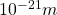or!!!!

In this brief note, I will explain you why this is so, in a simple way…

Typical scales of energy: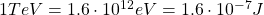We can use the Heisenberg uncertainty principle now: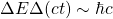OrWe plug the numbers,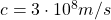,, and then we get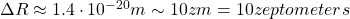Of course, this is a mere approximation. To be more precise, the LHC studies cross sections (as Rutherford, Geiger and Muller did but at higher energies. Therefore, it is more accurate to say that LHC measures interaction areas (or cross sections). How small are the area interactions that the LHC does explore?  The smallest cross section that the LHC can measure is about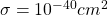. It means that the smallest effective distance that the LHC can reach isRemark: experimental physicists working at the LHC use an area/cross-section unit called barn., where 1fm is the fermi or femtometer.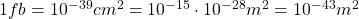The smallest cross-section that LHC (at 14TeV) could measure is more or less about 0.1fb.

We can compare to some known typically small distances:

1) Hydrogen atom smallest orbit in the Bohr model. The n=1 orbit (1s orbital in the quantum picture) for the electron in the Hydrogen atom is about 0.53 angstroms, or aboutor about 53 picometers!

2) The atomic nucleus and the effectic mesonic interaction (Yukawa like) appear at about 1 fm (1 femtometer, or 1 fermi). 1fm is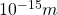. It is 1000 orders of magnitude smaller than the lowest orbit in the Bohr model of the hydrogen atom.

3) The weak interaction appears at an effective distance about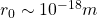, where the W and Z bosons arise as massive bosons (resonances). This distance is 1000 times smaller than the typical distance of atomic nuclei, and 1000000 times smaller than the lowest orbit in the hydrogen atom..

Therefore, the atomic nuclei are typically 1000 times smaller than “atoms” and about 1000 bigger than than the typical energy scale of electroweak gauge bosons. Curiously, the alledged Higgs-like boson is near the electroweak scale and it lives in a scale of lenght about 10-100 times bigger than the smallest scale of length we can explore with the LHC.

Question: Why the Higgs boson lives so close to the electroweak scale of energy/distance?

We don’t know yet. There is no symmetry in the SM protecting the Higgs particle (a boson) to become heavier (or very heavy indeed). The answer could be supersymmetry, or that the Higgs boson is secretly composed by smaller particles, or something more fantastic as the Higgs field itself being some kind of remnant from extra dimensions of space! The LHC was built in order to search for the Higgs field, study its properties AND to see a little bit beyond.

What will we find? Believe me, that is what makes Science so interesting!

Finally, let me point out that the typical Planck length is about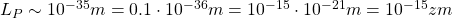That is, the Planck length is two far away yet from current colliders, about 15 orders of magnitude away!

Welcome to the LHC era, welcome to the Zeptospace!

Final remark: The LHC at 7TeV (full energy) per beam can accelerate protons up to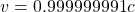i.e., up to 99.9999991% of the speed of light. The proof is easy using relativistic kinematicsUsing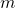as the proton massand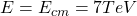, we getInserting here the above data, we check the previous claim, i.e.,See you in my next blog post!

This site uses Akismet to reduce spam. Learn how your comment data is processed.